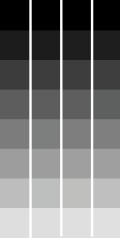# Linear Algebra - Scalar

Real number in linear algebra are called scalars and relates to vectors in a vector space through the operation of scalar multiplication.

Greek letters ($\alpha, \beta, \gamma$ ) denote scalars.

Discover MoreLinear Algebra - Vector

tuple in Linear algebra are called vector. A vector is a list of scalar (real number) used to represent a When the letters are in bold in a formula, it signifies that they're vectors, To represent...
Number System - Real Number (Scalar) -

A real number is a value that represents a quantity along a line. They have been called real to distinguish them from imaginary number. imaginary roots of polynomials The real numbers include all...